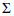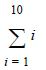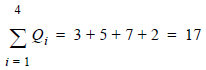# Summation

## Producer Field Guide

HGD_Product
Producer Field Guide
HGD_Portfolio_Suite
Producer

A commonly used notation throughout this and other discussions is(Sigma), used to denote a summation of values.

For example, the notationis the sum of all values of i, ranging from 1 to 10, which equals:

1 + 2 + 3 + 4 + 5 + 6 + 7 + 8 + 9 + 10 = 55.

Similarly, the value i may be a subscript, which denotes an ordered set of values. For example:Where:

Q1 = 3

Q2 = 5

Q3 = 7

Q4 = 2